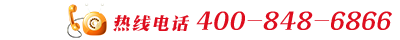# DJYJVP22计算机屏蔽电缆 定做

．聚乙烯绝缘聚氯乙烯护套计算机用屏蔽电缆(包括DCS电缆)
DJYV(R)P

DJYPV(R)

DJYPV(R)P

DJYV(R)P2

DJYP2V(R)

DJYP2V(R)P2

DJYV(R)P3

DJYP3V(R)

DJYP3V(R)P3

DJYVP(R)22

DJYP(R)V22

DJYPV(R)P22

DJYV(R)P2-22

DJYP2V(R)22

DJYP2V(R)P2-22

DJYVP3(R)-22

DJYP3V(R)22

DJYP3V(R)P3-22

2．聚氯乙烯绝缘聚氯乙烯护套计算机用屏蔽电缆(包括DCS电缆)
DJVV(R)P

DJVPV(R)

DJVPV(R)P

DJVV(R)P2

DJVP2V(R)

DJVP2V(R)P2

DJVV(R)P3

DJVP3V(R)

DJVP3V(R)P3

DJVV(R)P22

DJVPV(R)22

DJVPV(R)P22

DJVV(R)P2-22

DJVP2V(R)22

DJVP2V(R)P2-22

DJVV(R)P3-22

DJVP3V(R)22

DJVP3V(R)P3-22